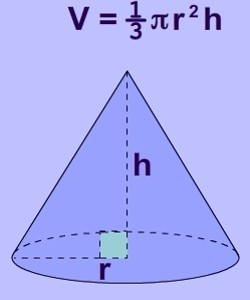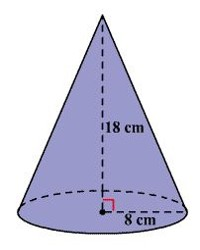Volume of a Cone

A cone is a three-dimensional figure with one circular base. A curved surface connects the base and the vertex. The volume of a 3 -dimensional solid is the amount of space it occupies.  Volume is measured in cubic units ( in3, ft3, cm3, m3 etc cetera).

The volume of a cone is: 1/3(Area of Base)(height) = 1/3 π r2 hThe volumes of a cone and a cylinder are related in the same way as the volumes of a pyramid and a prism are related. If the heights of a cone and a cylinder are equal, then the volume of the cylinder is three times as much as the volume of a cone.

Example:

Find the volume of the cone shown. Round to the nearest tenth of a cubic centimeter.Solution

From the figure, the radius of the cone is 8 cm and the height is 18 cm.

The formula for the volume of a cone is, V = 1/3 πr2 h

Substitute 8 for r and 18 for h.

V = 1/3 (3.1416) (8)2 (18)

≈ 1206.4

Therefore, the volume of the cone is about 1206.4 cubic centimeters.

Information Source: Varsity Tutors